# 16: Relative risks (Key Odd)

## Review Questions

1. Risk = the probability of an event occurs over a set period of time. (When an epidemiologist refers to risk they actually mean  average risk, incidence proportion, or cumulative incidence.) Relative risk =  the risk in one group relative to (divided by) that of another.
2. relative risk (risk ratio or prevalence ratio, depending on underlying frequency measure)
3. estimator =(also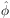); parameter = RR (also f )
4. 100% greater
5. When (a) disease is rare (risk < 5%), (b) duration of disease is the same in the exposed and non-exposed groups, and (c) disease does not influence exposure status differentially
6. less than 5.
7. R. A. Fisher
8. Continuity-corrected chi-square tests always produce larger (more conservative) P values.
9. Confounding is a bias in an association explained by extraneous variables.
10. An extraneous factor can confound an association by being associated with the explanatory factor and by being an independent predictor of the response.

16.1 Induction of labor

(A)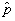1 = 1 / 111 = 0.0090, or 0.9% and2 = 13 / 117 = 0.1111, or 11.1%
(B)= 0.08. Induction was associated with a 92% reduction in risk.
(C) 95% confidence interval for RR:   ln= -2.526; se = 1.029; 95% CI for lnRR = -2.526 ± (1.96)(1.029) = -2.526 ± 2.017 = (-4.543, -0.509); 95% CI for RR  = (0.01, 0.60).

16.3 HIV infection among women entering the New York State Correctional System

(A)1 = 61 / 136 = 0.4485 [approx. 45%] and2 = 27 / 339 = 0.0796 [approx. 8%]

(B)= 0.4485 / 0.796 = 5.6315 @ 5.63. Interpretation: You could say prevalence in the IVDU+ group is 5.63 times that of the IVDU- group. You could also say prevalence is 463% greater with IVDU+. Some of you may be tempted to say there is a strong positive association between IVDU and HIV. This is OK as long as you also quantify the relation in one form or an other. Relation between prevalence ratios and risk ratios: Prevalence ratios are equal to risk ratio when certain assumptions are met. The assumptions are: (a) duration of disease is similar in the groups, (b) disease is rare, and (c) disease does not influence the exposure (Rothman and Greenland, 1998, p. 64). In the current problem, the disease is not rare, so the prevalence ratio is not a risk ratio.

(C) 95% confidence interval: ln= ln(5.6315) = 1.728; selnf^ = 0.208; 95% CI for lnf  = 1.728 ± (1.96)(0.208) = (1.321, 2.137); 95% CI for f = e(1.321, 2.137) = (3.75, 8.47). This allows us to say with 95% confidence that the prevalence ratio parameter lies between 3.75 and 8.47. Some epidemiologists would interpret these limits as a "best-case scenario / "worst-case scenario."  In addition, the confidence interval "length" is a direct measure of the precision of the estimate. In any event, we say confidently that the prevalence ratio is between 3.75 and 8.47. Of course, this statement assumes data are valid.

(D) H0: RR = 1 versus H1: RR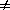1;  Expected frequencies (below) exceed 5, making Fisher's test unnecessary; Yates' chi-square = 85.08 with 1 df, P = 0.0000. The association is significant (Reject H0). Calculations shown below:

D+        D-
E+      25.196   110.804    136
E-      62.804   276.196    339
88       387        475

c2Yates' =  (|61 - 25.196| - 0.5)2 / 25.196   +   (|75 - 110.804| - 0.5)2 / 110.804  +
(|27 -
62.804| - 0.5)2 / 62.804   +   (|312 - 276.196| - 0.5)2 / 276.196
=  49.468                       +    11.249                  +
19.846                       +     4.513
=  85.075

(E) SPSS output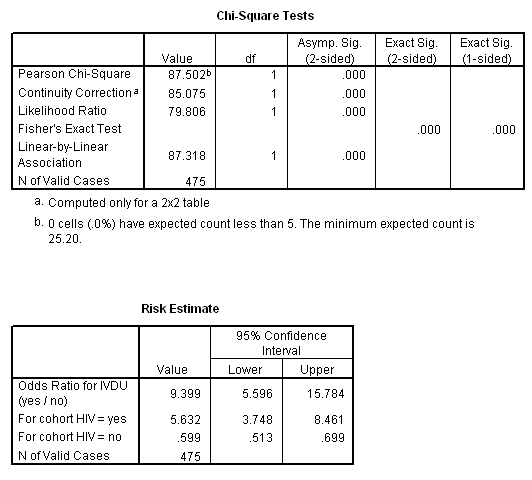(F) Sample size requirements: I used WinPepi (below) and assumed p2 = 0.08 (based on2 above). To detect an RR of  2, n1 = n2 = 489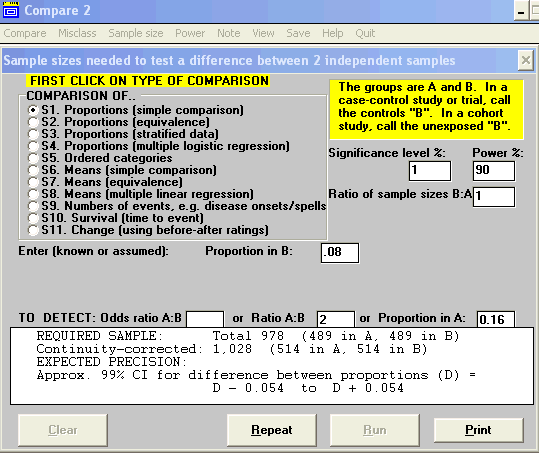(G) To detect an RR of  1.5, n1 = n2 = 1663

16.5 Cytomegalovirus and coronary restenosis.

(A)= (21/49) / (2/26) = 0.4286 / 0.0769 = 5.571. Risk in the CMV+ group is 5 to 6 times that of the CMV- group
ln= ln(5.571) = 1.717; SE = 0.6992; 95% confidence interval = e (0.3486, 3.0894) = (1.42, 21.93).
Interpretation: Several points should be made. The magnitude of the risk ratio should be noted (i.e., The imprecision of the estimate (broad confidence interval) should also be noted. It is also important to note that data support the CMV / restenosis hypothesis.

(B) X2stat, c = 8.29, df = 1, P = 0.0040; significant evidence against H0.

16.7 Oral contraceptives and myocardial infarction

(A) 2-by-2 table

HD+  HD-
OC+    13  4987   5000
OC-     7  9993  10000

(B)= (13/5000) /(7/10000) = 3.714; SE = 0.468; 95% confidence interval for the lnf = ln(3.714) ± (1.96)(0.468) = (0.395, 2.229); 95% confidence interval for f = e(0.395, 2.229) = (1.48, 9.30). The risk ratio point estimate is suggestive of almost 4 times the risk in the oral contraceptive group. The broad confidence interval suggests this estimate is not very precise.

(C) H0:RR = 1 versus  H1: RR1; X2stat  c-c = 7.67, df = 1; P = 0.0056. The evidence against H0 is significant

16.9 Kayexelate and colonic necrosis

(A) Determine the incidence of colonic necrosis in the groups.1 = 2 / 117 = 0.017;2 = 0 / 862 = 0.000

(B) Calculate expected cell counts. [Below] Which test would you use with these data? Fisher's exact or the Mid-P exact

 Expected Necrosis + Necrosis - Generic + 0.24 116.67 Generic - 1.76 860.24

(C) Calculate and report an appropriate P value.  Calculated with www.OpenEpi.com: Fisher's P (two-sided) = 0.028; Mid-P P = 0.014

16.11 Contingency tables involving small numbers (3-by2)

(A) You cannot use a chi-square test because two of the table cells have expected values that are less than 5 (see below).

 Expected Normal teeth Malocclusion 1.739 18.261 Bottle fed 1.913 20.087 Brst +bottle 4.348 45.652

(B) Fisher's P = 0.1503; Mid-P P = 0.1390
[Advanced users: X2stat = 4.154; df = 2, P = 0.125, which is not too different from the Mid-P value. Here's another interesting fact: only 8 of 92 subjects (9%) had normal teeth--perhaps the Austin Power's movies were not exaggerating the state of British dental health in the 60s!]

16.13 Don't sweat the small stuff or P = 0.05.  X2stat = 4.107, df = 1; P = 0.043; X2stat, c = 3.598, df = 1; P = 0.058. Although the regular chi-square statistics produces a P value that is a little below 0.05 and the continuity-corrected chi-square statistic produces a P-value that is a little above 0.05, it would not be reasonable to derive different conclusions from the same data. There is nothing sacred about either of these test statistics or alpha = 0.05. Both tests provide fairly good evidence against the null hypothesis.

16.15 Do seatbelt laws prevent injury? Display the data in a 2-by-2 table [below] and compare the incidence of "no injury" to injury" in the form of a risk ratio and interpret these results. RR = 1.023, indicating a 2.3% increase in the "no injury" rate.  Include a 95% confidence interval... (1.006, 1.04)

 Injury No Yes Total After 1281 103 1384 Prior 6596 694 7290 Total 7877 797 8674

16.17 Anger and heart disease (hard outcome, hypertensives).

Calculate risk ratios for
(A) moderate-anger vs. low-anger 0.83
(B)
high-anger vs. low-anger. 1.01
(C)
Does a dose-response pattern seem to emerge? No